## Demonstration

A quick demonstration of capping the lines.

First, load the package, generate a dataset and display it.

``````library(ggplot2)
``````
``````## Registered S3 methods overwritten by 'ggplot2':
##   method         from
##   [.quosures     rlang
##   c.quosures     rlang
##   print.quosures rlang
``````
``````## Registered S3 method overwritten by 'dplyr':
##   method               from
##   as.data.frame.tbl_df tibble
``````
``````library(lemon)

dat1 <- data.frame(
gp = factor(rep(letters[1:3], each = 10)),
y = rnorm(30),
cl = sample.int(3, 30, replace=TRUE),
cl2 = sample(c('a','b','c'), 30, replace=TRUE)
)

my.theme <- theme_light()

(
p <- ggplot(dat1, aes(gp, y)) + geom_point() + my.theme
)
``````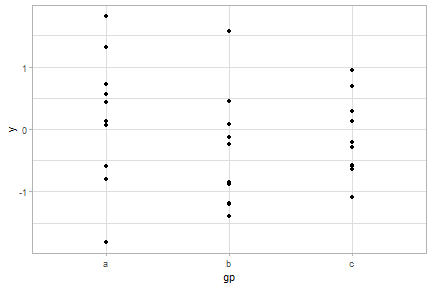NB: In order to manipulate the axis lines, they must be drawn. Modify the theme so the `panel.border` is not drawn (it will be on top of the axis lines), and have the axis lines drawn:

``````my.theme <- my.theme + theme(panel.border=element_blank(),
axis.line = element_line(),
axis.ticks = element_line(colour='black'))
p <- p + my.theme
``````

Now, let's have some fun.

We cap the bottom axis line to the right-most tick. The left end is also capped by the amount specified with the `gap` argument (at time of writing, defaulted at `0.01`).

``````p + coord_capped_cart(bottom='right')
``````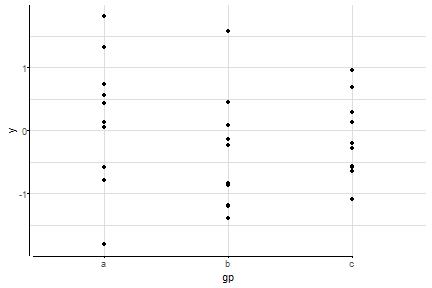To keep the axis lines consistent, we also specify the `left` argument, which still caps the left axis line by the amount specified with the `gap` argument.

``````p + coord_capped_cart(bottom='right', left='none')
``````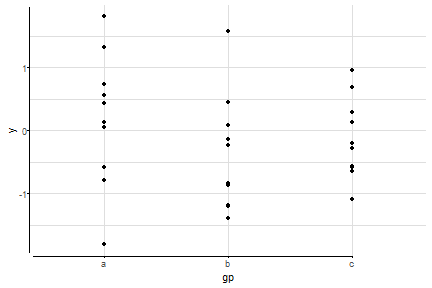To avoid overplotting, we can apply a jitter. To emphasise that the x-axis is categorical, we can place brackets. We finally polish the plot by removing the redundant vertical grid lines.

``````ggplot(dat1, aes(gp, y)) + geom_point(position=position_jitter(width=0.2, height=0)) +
coord_capped_cart(left='none', bottom=brackets_horizontal()) +
my.theme + theme(panel.grid.major.x = element_blank())
``````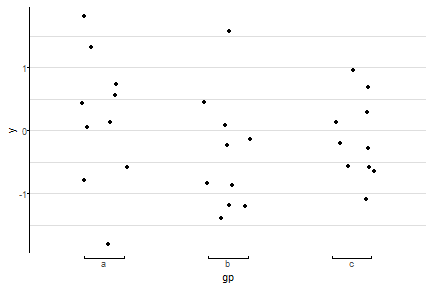## The `coord` objects

ggplot2's Cartesian coordinates systems, `coord_cartesian`, have been extended to allow for flexible specification of how axes are drawn. You've seen them above. The following table summarises the connection between ggplot2's coord functions and those of lemon.

ggplot2 lemon's flexible lemon's short hand
`coord_cartesian` `coord_flex_cart` `coord_capped_cart`
`coord_flip` `coord_flex_flip` `coord_capped_flip`
`coord_fixed` `coord_flex_fixed`

The short hand functions in the table's right column simply are almost identical to those in the middle column. If one of the side arguments are specified with a character value, the relevant axis drawing function is used. You can however choose to use e.g. `brackets_horizontal` in place.

### The axis drawing functions

The functions `capped_horizontal` or `brackets_vertical` returns a function that is called when ggplot2 prints the plot. In this package, we use ggplot2 to build the axes, then modify in place the return grobs.

The function is called by the `coord` objects when printing the plot, and is called with the arguments

``````scale_details, axis, scale, position, theme
``````

The function should then return a grob. Some pointers to how it is used can be found in ggplot2's help pages on ggproto (`?"ggplot2-ggproto"`)

The arguments are as follows[tip_rds]

[tip_rds]: To get the contents of the arguments, I usually include a line in the function's code to save them as a RDS object: `saveRDS(list(scale_details=scale_details, axis=axis, scale=scale, position=position, theme=theme), file='whatever.rds')`

`scale_details`: Details of the scales in 'npc' units (see `grid::unit`). In the example below, a secondary y-axis is used, so we find both `y.major`, etc., and `y.sec.major`, etc.

``````List of 20
\$ x.range           : num [1:2] 0.4 3.6
\$ x.labels          : chr [1:3] "a" "b" "c"
\$ x.major           : num [1:3] 0.187 0.5 0.812
\$ x.minor           : NULL
\$ x.major_source    : int [1:3] 1 2 3
\$ x.minor_source    : NULL
\$ x.arrange         : chr [1:2] "secondary" "primary"
\$ y.range           : num [1:2] -2.17 2.26
\$ y.labels          : chr [1:5] "-2" "-1" "0" "1" ...
\$ y.major           : num [1:5] 0.0378 0.2635 0.4892 0.7149 0.9406
\$ y.minor           : num [1:9] 0.0378 0.1506 0.2635 0.3763 0.4892 ...
\$ y.major_source    : num [1:5] -2 -1 0 1 2
\$ y.minor_source    : num [1:9] -2 -1.5 -1 -0.5 0 0.5 1 1.5 2
\$ y.sec.range       : num [1:2] -10.8 11.3
\$ y.sec.labels      : chr [1:5] "-10" "-5" "0" "5" ...
\$ y.sec.major       : num [1:5] 0.038 0.263 0.489 0.715 0.941
\$ y.sec.minor       : num [1:9] 0.0378 0.1506 0.2635 0.3763 0.4892 ...
\$ y.sec.major_source: num [1:5] -10 -5 0 5 10
\$ y.sec.minor_source: num [1:9] -10 -7.5 -5 -2.5 0 2.5 5 7.5 10
\$ y.arrange         : chr [1:2] "primary" "secondary"
``````

The secondary y-axis mulitplied the values by 5. Ticks are drawn at `major` coordinates, as are major grid lines. Minor grid lines, if the theme supports them, are drawn at `minor`. Observe the connection between `y.label` and `y.major_source`; it only becomes apparent when also observing the same for the x-axis. The `x.major_source` are the factor integers.

`axis`: Character, either `"primary"` or `"secondary"`. Recall that the function is called per axis drawn.

`scale`: Character, either `"x"` or `"y"`.

`position`: Character, one of the sides, `"top"`, `"right"`, `"bottom"`, or `"left"`.

`theme`: The plot's theme. This is however not in absolute terms, e.g. so text sizes may be described in relative terms to the base size. To resolve a usable `gp` (graphic parameters, see `?grid::gpar`) for a grob, use `ggplot2:::element_render(theme, 'axis.text.x')`, in which the second argument would resolve to the labels of the x-axis.

### Brackets

The brackets comes in two orientations: `brackets_horizontal` and `brackets_vertical`. If you attempt to use a vertical bracket on a horizontal axis, it will fail with a undescriptive error.

The bracket functions accept a `direction` argument, which can be used to control which direction the end-points are pointing:

``````p <- ggplot(mpg, aes(cyl, hwy, colour=class)) +
geom_point(position=position_jitter(width=0.3)) +
scale_x_continuous(breaks=c(4,5,6,8), sec.axis=dup_axis()) +
scale_y_continuous(sec.axis=dup_axis()) +
coord_flex_cart(bottom=brackets_horizontal(),
top=brackets_horizontal(direction='down'),
left=brackets_vertical(),
right=brackets_vertical(direction='right')) +
my.theme
p
``````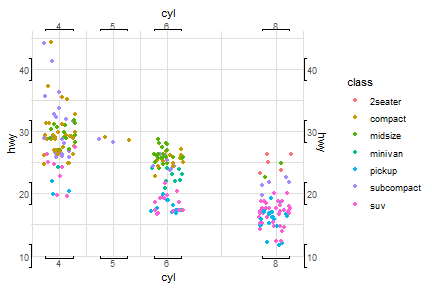The look of the brackets are controlled via `theme(axis.ticks)`. The length of the end-point are controlled via the theme `theme(axis.ticks.length)`. If these needs to be specified for each margin, use the argument `tick.length`:

``````p <- ggplot(mpg, aes(cyl, hwy, colour=class)) +
geom_point(position=position_jitter(width=0.3)) +
coord_flex_cart(bottom=brackets_horizontal(tick.length=0),
left=brackets_vertical(tick.length = unit(1, 'cm'))) +
my.theme
p
``````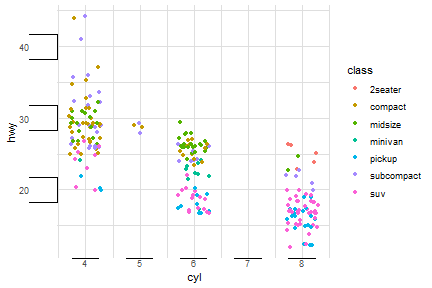As shown above, using `tick.length=unit(0, 'cm')` results in a flat line.

## Facets

Having produced such wonderous axes, it is a pity they are not plotted around all panels when using faceting.

``````dsamp <- diamonds[sample(nrow(diamonds), 1000), ]
(d <- ggplot(dsamp, aes(carat, price)) +
geom_point(aes(colour = clarity)) +
coord_capped_cart(bottom='none', left='bottom') +
facet_grid(.~cut) + my.theme
)
``````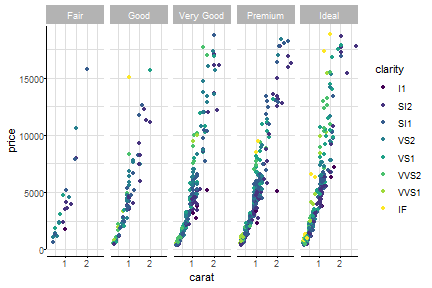`facet_grid` and `facet_wrap` have been implemented in versions that display the axis lines (and labels) on all panels. They work exactly like ggplot2's functions, and are named with `_rep_`:

``````d + facet_rep_grid(.~cut)
``````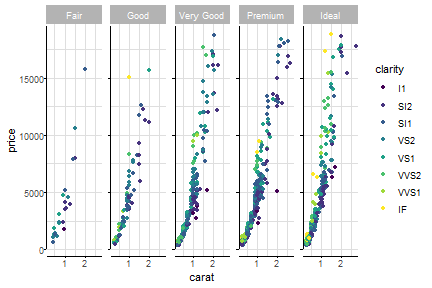If we want the labels shown as well, use the argument:

``````d + facet_rep_grid(.~cut, repeat.tick.labels = TRUE)
``````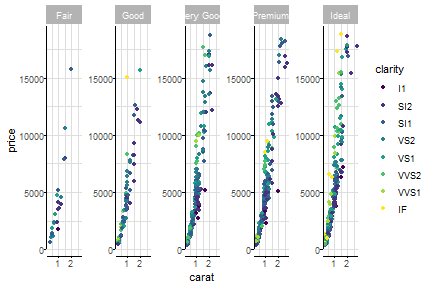It also works for `facet_wrap`:

``````(d2 <- d + facet_rep_wrap(~cut, ncol=2))
``````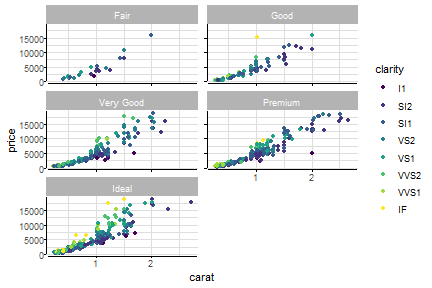Finally, the legend can be repositioned to fit in a panel by using `reposition_legend`. See the vignette `legend`. The addition of `theme(legend.background)` is merely to provide a border around the legend.

``````d2 <- d2 + guides(colour=guide_legend(ncol=3)) +
theme(legend.background = element_rect(colour='grey'))
reposition_legend(d2, position='center', panel='panel-2-3')
``````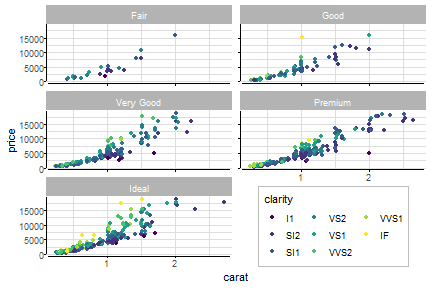## Scales

As of lemon v0.4.2, you can now use symmetric y- og x-axis scales.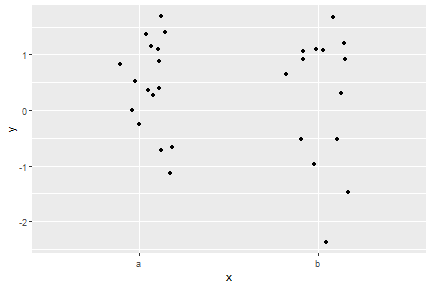``````p + scale_y_symmetric(mid=0)
``````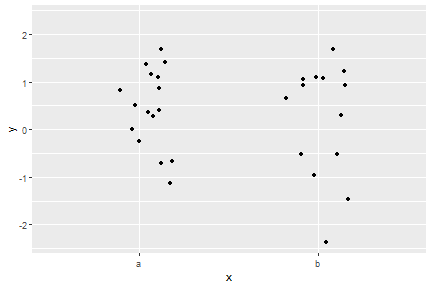The same effect could be achieved with `coord_cartesian` or use of `scale_y_continuous`; however when used with a facet where each row (or column) should scale to the data, `scale_x_symmetric` and `scale_y_symmetric` ensures the data will remain centered.